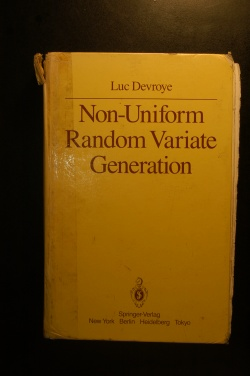Home Newsletter News BooksLanguage/LinguaBooks of Mathematics· Book News · Most clicked · Least clicked · Books Index · Search on Amazon

Search for a Book
Add a new BookProbability and Statistics

 Mathematics -> Probability and Statistics Search on Amazon

 Generalized Linear Models Large Deviations Percolation Probability Theory Statistics Stochastic Processes Time series analysis

Non-Uniform Random Variate Generation
Author: Luc Devroye  Language:This text Is about one small field on the crossroads of statistics, operations research and computer science. Statisticians need random number generators to test and compare estimators before using t . . . . .
Foundations of Descriptive and Inferential Statistics
Author: Henk van Elst   Language:These lecture notes were written with the aim to provide an accessible though technically solid introduction to the logic of systematical analyses of statistical data to undergraduate and postgraduate . . . . .
Introduction to Probability and Statistics
Author: Prof. Dmitry Panchenko  Language:Contents: Probability, Set Operations; Properties of Probability; Finite Sample Spaces, Some Combinatorics; Multinomial Coefficients, Union of Events; Matching Problem, Conditional Probability; Indep . . . . .
Lecture Notes on Free Probability
Author: Vladislav Kargin  Language:Lecture notes for a graduate course lectured at Stanford University.
Foundations of the Theory of Probability
Author: Kolmogorov  Language:The book "Kolmogorov: Foundations of the Theory of Probability" by Andrey Nikolaevich Kolmogorov is historically very important. It is the foundation of modern probability theory. The monograph appear . . . . .
Lectures on Self-Avoiding Walks
Author: Roland Bauerschmidt, Hugo Duminil-Copin, Jesse Goodman, Gordon   Language:These lecture notes provide a rapid introduction to a number of rigorous results on self-avoiding walks, with emphasis on the critical behaviour. Following an introductory overview of the central pro . . . . .
Fundamentals of probability
Author: Marco Taboga  Language:Contents: Fundamentals of probability; Probability and probability measures; Zero-probability events; Conditional probability; Independent events; Random variables and univariate probability distribut . . . . .
Probability and Statistics Cookbook
Author: Matthias Vallentin  Language:This cookbook integrates a variety of topics in probability theory and statistics. It is based on literature [1, 6, 3] and in-class material from courses of the statistics department at the Univalle . . . . .
Electronic Statistics Textbook
Author:   Language:This Electronic Statistics Textbook offers training in the understanding and application of statistics. The material was developed at the StatSoft R&D department based on many years of teaching underg . . . . .
Probability and statistics EBook
Author: UCLA and others  Language:Contents: Introduction to Statistics; Describing, Exploring, and Comparing Data; Probability; Probability Distributions; Normal Probability Distribution; Relations Between Distributions; Point and Int . . . . .

Add a new book
```Home |  Authors | About | Contact Us |  Email